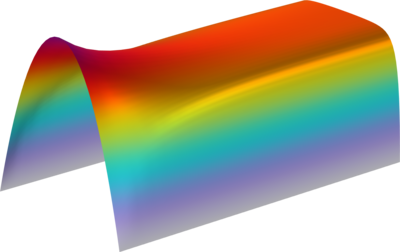# Application Gallery

## Hartmann Boundary Layer

Application ID: 73451

Classical MHD benchmark problem was solved analytically by J. Hartmann. He considered laminar incompressible flow between two planes (in planar duct) in transversal imposed magnetic field under next assumptions: * fully developed flow (far from inlet) * fluid properties are constant * the distance between planes is equal to 2d * average inlet velocity is U0 * imposed magnetic field is H0. This model solves this problem numerically using dimensional equations and then comparison with analytical dimensionless solution of Hartmann is made.This model example illustrates applications of this type that would nominally be built using the following products: